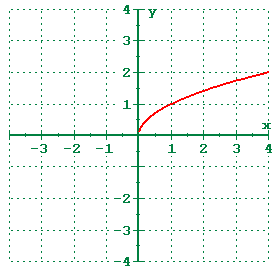# Unit 1 Practice Test

12 Questions | Total Attempts: 241SettingsCreate your own QuizThis is a practice test for Mrs. Rhinehart, Mrs. Rucker and Mrs. Gee's Math1 Unit 1 Test.

• 1.
Given f(x) = -2x2 , evaluate f(-3).
• A.

18

• B.

36

• C.

-18

• D.

-36

• 2.
The function c(m) = .25m + 10, represents the total monthly cost for a cell phone, where m represents the number of minutes used.  What is the dependent quantity?
• A.

Number of Minutes

• B.

Total monthly cost

• C.

Cost per minute

• 3.
This function is a graph of which of the following types of functions?
• A.

Linear

• B.

Absolute Value

• C.

• D.

Cubic

• E.

Square Root

• 4.
Describe the transformations from the parent function to h(x) = -2(x - 3)3 .
• A.

Shift down, Stretch, then a Reflection across the y-axis

• B.

Shift right, Shrink, then a Reflection across the x-axis

• C.

Shift left, Shrink, then a Reflection across the y-axis

• D.

Shift right, Stretch, then a Reflection across the x-axis

• 5.
Which of the functions below is the correct function for the parent linear function that has been reflected across the y-axis and shifted up 5?
• A.

F(x) = x + 5

• B.

F(x) = -x + 5

• C.

F(x) = -x - 5

• D.

F(x) = x -5

• 6.
Which of the following is the correct description for the end behavior of f(x) = -(x)2 + 3?
• A.

Both arms reach up

• B.

Both arms reach down

• C.

Right arm reaches up, Left arm reaches down

• D.

Right arm reaches down, Left arm reaches up

• E.

One arm reaches right

• 7.
A set of functions with similar characteristics is the definition of . . .
• A.

Parent Function

• B.

Domain

• C.

Family of Functions

• D.

Range

• 8.
True/False:  The function f(x) = x3 has a maximum at (0, 0).
• A.

True

• B.

False

• 9.
Given the sequence 0, 1, 3, 6, 10, . . ., find S(7).
• A.

21

• B.

15

• C.

7

• D.

0

• 10.
Using the table below, find the rate of change when x changes from -2 to 1.x           -2         -1        0         1         2f(x)        -6          1        2         3        10
• A.

-3

• B.

1/3

• C.

-1/3

• D.

3

• 11.
The function g(x) = 10x represents the total profit from selling t-shirts, where x is the number of t-shirts sold.  Find the rate of change when the number of t-shirts increases from 3 to 10.
• A.

7

• B.

10

• C.

70

• D.

5

• 12.
Which of the following is the correct slope and y-intercept for the linear function f(x) = -5x + 8?
• A.

Slope = -5, y-intercept = 8

• B.

Slope = 8, y-intercept = -5

• C.

Slope = 5, y-intercept = 8

• D.

Slope = -8, y-intercept = 5

Related TopicsBack to top
×

Wait!
Here's an interesting quiz for you.